# flp

/ 0评 / 0

FLP 这篇论文在分布式领域有着重要的作用，当然，这篇文章也写得晦涩难懂。这是第一篇我死扣每个字读下来的分布式论文，十分吃力，在此记录下，并且竟可能写的简单，希望能够帮助初入分布式计算的新人们够更加容易理解 FLP 论文。当然再怎么简单，数学的符号是跑不了的，但是不要害怕，一个一个字看下来即可。

-- 写于 2021.12.12

## 前言

FLP 定理相当于给分布式一致性算法订上了棺材板，它证明了根本不可能实现一个真正意义上的共识算法。

FLP 证明了在一个异步的网络环境中（没有丢包，消息不会被重复传递），最多只有一个节点发生故障，都不存在一个共识算法。可以想象如此宽松的条件下都不存在，更不用说现实环境了。

Validity：有效性（合法性），如果所有节点中的数据只有 0 和 1 两种，那么最后达成一致的决议一定是 0 和 1 这两种的一个。不可能说算法莫名其妙的达成一个一致的数值如 -1。

Agreement：一致性，所有节点达成一致的决议。

Termination：终止性，所有正常运行的节点最终都能够做出决议。

## Terminology 术语

Consensus algorithm：共识算法，也可以说是一致性算法，比如 Raft，Paxos 都可以叫 consensus algorithm。

Byzantine Generals problem：拜占庭将军问题，就是指数据在传输的过程中被篡改了怎么办。通常 consensus algorithm 都会假设不出现拜占庭将军问题，即假设了数据在传输过程中不会被篡改（节点不会收到一个被伪造过的数据）。因为通常情况下，网络协议如 TCP 就能够保证你拿到的数据大概率是对的，而且一致性算法一般运行在内网里面，相对安全。

Process：在论文中你可以把它理解为分布式系统中的一个节点。每一个 process $p$ 有一个 input register $x_p$，可以理解为输入值，只有 0，1两种。此外 process p 还有一个 output register $y_b$ ，$y_b$ 的值有 b，0，1 三种 。$x_p \in {0,1},y_p \in {b,0,1}$。

Message system：把这个想象成一个网络就行了，连接了整个集群中的所有 process。整个系统中所有的 process 均共用这一个 message system，process 通过这个进行相互之间的通讯，就是互相发送 message。

Asynchronous system：异步系统。论文中对 asynchronous system 做了如下要求：（1）你不知道每一个 process 的相对处理速度，也就是你不知道哪一个 process 会先处理完。（2）Message system 中的 message 可能会延迟到达（delay，而且你不知道延迟多久，但一定会达到）。（3）整个系统中不存在公用一个同步的时钟（synchronized clock），这意味着你不能通过时间来设计算法。（4）你没有能力侦测到某一个 process 的 dead（崩溃），某一个 process 在 dead 前也不会提前通知其他 process。

Nonfaulty：无故障。

Internal state：一个 process 的 input register $x_p$，output register $y_b$，内部存储空间等等构成一个 process 的 internal state。

Configuration：一个 Configuration 包含了整个集群中所有 process 的 internal state 外加 message system。

Step：从一个 configuration 到另外一个 configuration 的过程叫 step。比如一个 process 从 message system 接收了一份数据，然后他自己改变了自己的 output register，发送了一个 message 到 message system 中，从而整个系统形成了一个新的 configuration。

Atomic step：一个原子的 step，这一步 step 包含了 process 从 message system 接收数据，本地处理，通过 message system 发送数据给别的 process 这一过程。

Decision state：达成决议的状态，比如该 process 达成了决议，确定自己的值为 0 或 1。

Initial state：所有 process 除了 input register 可以不同，其它值都是固定给死的，其中 output register $y_b=b$。

Initial configuration：所有 process 都为 initial state，而且 message system 为空。Run：一个 run 包含多个 step。

Deciding run：在这个 run 中，如果某些 process 能够到达 decision state，那么这个 run 就叫 deciding run。（注意，论文里面要求某些 process 即可）。

Admissible run：最多只有一个 process dead，其余正常的 process 都从 message system 中接收到属于它自己的 message（message system 中所有 message 发完）。

Event：$e(p,m)$ 表示 process $p$ 收到数据 $m$ 并进行处理这一过程。

Schedule：一系列的 event 组成一个 schedule。schedule 用 $\sigma$ 表示。我们用 $\sigma(C)$ 表示 configuration $C$ 通过 schedule $\sigma$ 后得到的结果。同时说明 $\sigma(C)$ 是从 $C$ reachable（可达的）。如一个 configuration 可以从某些 initial configuration reachable，那么此 configuration 是 accessible（accessible 其实和 reachable 意思差不多，只不过换个单词区分一个从 initial configuration 变的，一个从 configuration 变的）。

Accessible configuration：见 schedule 里面的说明。

Decision value：如果在一个 configuration $C$ 中，有些 process 已经处于 decision state，其输出值 $y_b=v$ ，那么我们就认为这个 configuration 的 decision value 为 $v$。注意：这里同样不需要所有 process 都处于 decision state，因为论文放宽了条件，只要一部分 process 能够做出决议，就算整体做出了决议。

Partially correct：符合下面两个条件就可以叫 partially correct。（1）所有 no accessible configuration 都包含两个及以上的 decision value。相当于变相的说 所有 accessible configuration 都只有一个 decision value。直白的说，就是整个集群中所有 process，要么你还没处于 decision state，而处于 decision state 的 process，其达成决议的值都是一样的。（2）假设 decision value $v\in {0,1}$ 。存在一些 accessible configuration 有 decision value $v$。注意只是有些，因为有些 accessible configuration 可能还做不出 decision value。

Totally correct：在只有一个 process dead 的情况下，其是 partially correct，同时每一个 admissible run 都是 deciding run。那它就是 totally correct。

Bivalent：如果 configuration 中包含两个 decision value（即多个 process 中，有些 decision state 为 0，有些为 1），那就叫 bivalent。

Univalent：如果 configuration 中只有一个 decision value，那就叫 univalent。

0-valent：是 univalent，其 decision value 为 0。

1-valent：是 univalent，其 decision value 为 1。

## Model

1. 不考虑 Byzantine failures。
2. 假设 message system 是可靠的不会丢失数据，不会给 process 重复发送数据（exactly once）。但是注意，message system 里面发送的数据可以是乱序的，即不保证数据传输的先后顺序，而且有时候会返回空（ $\emptyset$）。

1. 为了简化，论文假设所有 process 的 input register $x_p \in {0,1}$ 。所有 nonfaulty process 都会在进入 decision state 时决议出一个值 output register $y_p \in {0,1}$。正常来说，既然是 consensus algorithm，那肯定要保证所有 nonfaulty process 在 decision state 选择的都是同一个值，比如大家都选 0 或 1。但是论文中在此处再一次放宽条件，它只要求部分 nonfaulty process 都够做出决定，到达 decision state 就行了。
2. 前面说过，message system 可能会返回 $\emptyset$ ，但是论文假设了，如果一个 process 不停的从 message system 接收 message，那它终究能够接收到本应该发给它的 message。这样就模拟了网络的延迟，但是消息一定会送达。（message system 通过发送 $\emptyset$ 的方式来模拟延迟）。

Consensus protocol $P$

Process 之间的通讯通过 message system 实现。message 的格式为$(p,m)$ ，$p$ 是目标 process，$m$ 是 message 内容。整个 message system 你可以想象成一个缓冲区 buffer（不保证 message 顺序）。

Message system 支持两种操作：

• $send(p,m)$，将 $(p,m)$ 放在 message system buffer 中。

• $receive(p)$ ，从 message system 中取出属于 process $p$ 的 message。如果取出成功，那 message system 就会移除这个 message。当然也有可能取出失败，返回一个 $\emptyset$ 。

Message system 通过上面两种操作，模拟了整个分布式系统中网络的不确定性（不过至少还保证了数据不会没了，比现实生活中好多了）。

## Lemma1 "Commutativity" property of schedules

Suppose that from some configuration $C$, the schedules $\sigma_1$ , $\sigma_2$ lead to configuration $C_1$, $C_2$ , respectively. If the sets of processes taking steps in $\sigma_1$ and $\sigma_2$, respectively, are disjoint, then $\sigma_2$ can be applied to $C_1$ and $\sigma_1$ can be applied to $C_2$ , and both lead to the same configuration $C_3$ 。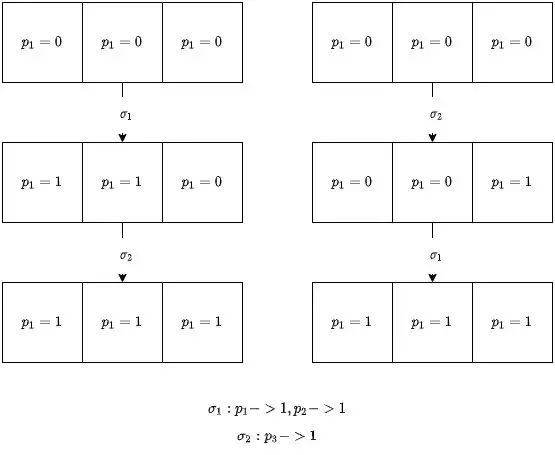## Main Result

No consensus protocol is totally correct in spite of one fault.

## Lemma2

$P$ has a bivalent initial configuration.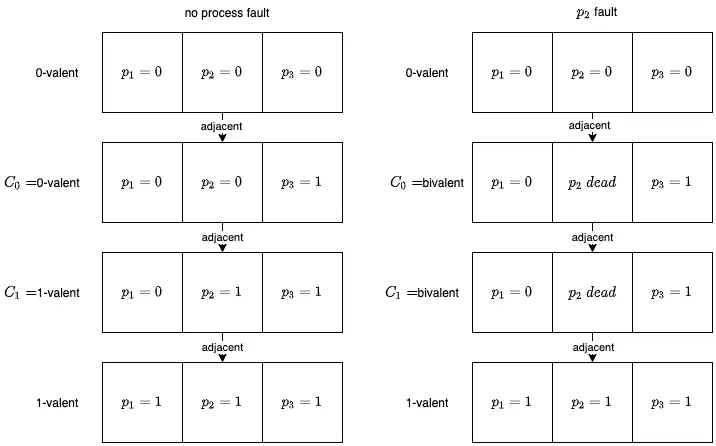## Lemma3

Let $C$ be a bivalent configuration of $P$, and let $e=(p,m)$ be an event that is applicable to $C$. Let $\mathbb{C}$ be the set of configurations reachable from $C$ without applying $e$, and let $\mathbb{D}=e(\mathbb{C})={e(E)|E\in \mathbb{C}}$ and $e$ is applicable to $E$. Then, $\mathbb{D}$ contains a bivalent configuration.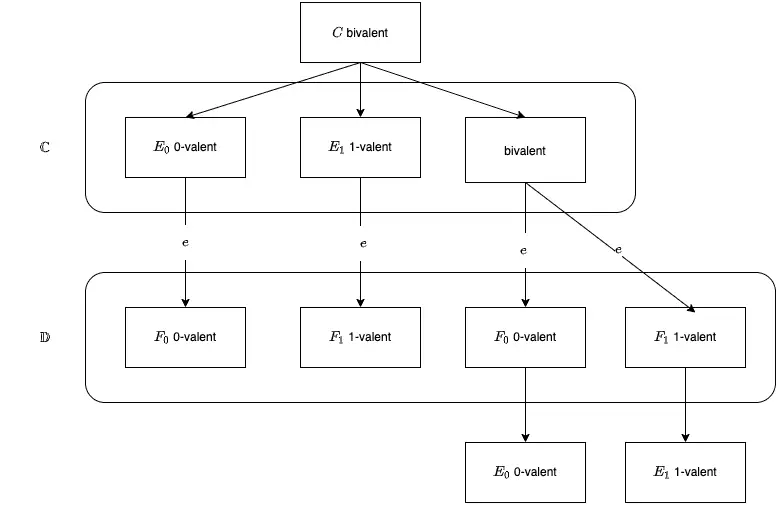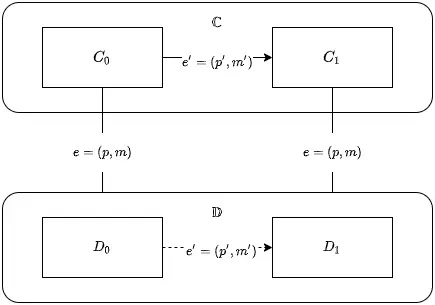Case 1： 如果 $p' \neq p$ ，根据 Lemma1 交换律，那么存在 $D_1=e'(D_0)$ ，因为 $e$ 和 $e'$ 没有交集，可以使用交换律。可以发现这样违背了 $D_i$ 是 univalent 的假设，你从 $D_0$ 的 0-valent 变成 $D_1$ 的 1-valent，那不是变相的说明了 $D_0$ 是 univalent 的。

Case 2： 如果 $p'=p$ ，有交集，不能用交换律了。这时我们考虑，存在一个 deciding run 从 $C_0$ 出发，在这个 deciding run 中，我们假设 $p$ 是 dead 的。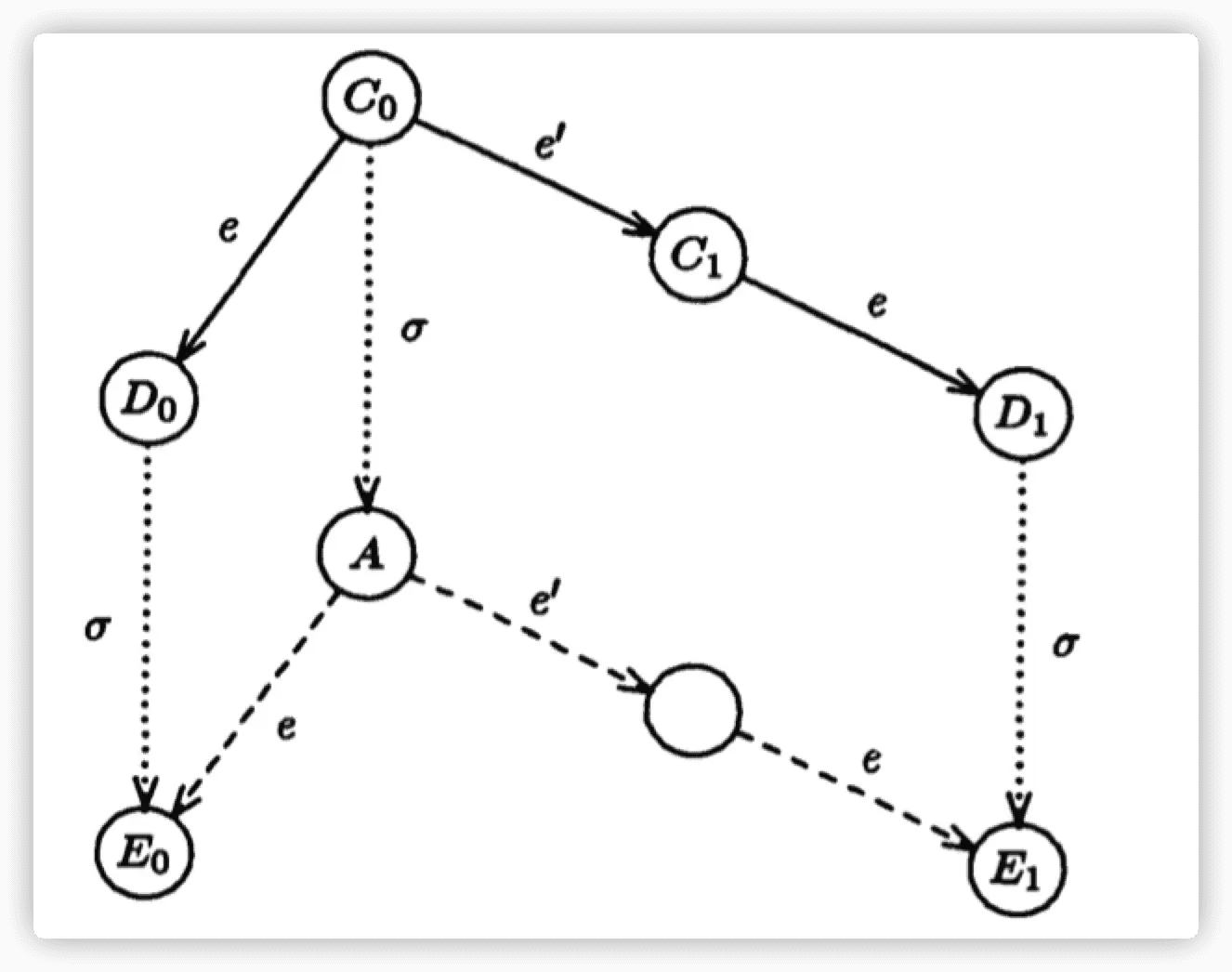## 参考文献

https://www.the-paper-trail.org/post/2008-08-13-a-brief-tour-of-flp-impossibility/

http://resources.mpi-inf.mpg.de/departments/d1/teaching/ws14/ToDS/script/flp.pdf

https://www.cnblogs.com/firstdream/p/6585923.html

https://danielw.cn/FLP-proof

https://blog.csdn.net/chen77716/article/details/27963079

https://zhuanlan.zhihu.com/p/36325917

http://loopjump.com/flp_proof_note/

https://www.jianshu.com/p/33b55df03cbd

http://www.cxyzjd.com/article/bucuo12345/85006533

• 默认
• 护眼
• 夜晚
• Serif
• Sans